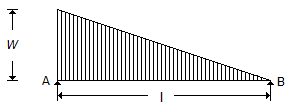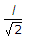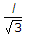# Mechanical Engineering - Strength of Materials - Discussion

Discussion Forum : Strength of Materials - Section 1 (Q.No. 33)
33.
The maximum bending moment for the beam shown in the below figure, lies at a distance of __________ from the end B.l/2
l/3Explanation:
No answer description is available. Let's discuss.
Discussion:
18 comments Page 1 of 2.

Salahuddin said:   5 months ago
No need to solve the bending moment equation.

BM is max where SF is zero. Therefore take a section x-x at a distance x from end B. Write SF equation and equate it to zero.

Thank you.
(2)

Sweetu said:   6 years ago
Bending moment will max. Where s.f is zero.
Take distance X m from B.
Nd s.f. = (wl/6)-(wX*X/2)=0.
So X=l/√3.
(1)

Vikki said:   6 years ago
Bending moment will maximum where shear force is zero.
We have shear for at dist X from point A is,
F= WL/6-[Wx^2/(2L)]. Since F=0.
WL/6=Wx^2/(2L),
On simplification we get x=L/√3.
(1)

Vikesh said:   6 years ago
How you got -WX?
In equation (WL/3)-Wx+[ Wx^2/(2L)].
Here WL/3 is Ra.
And Wx^2/(2L) for UVL.
Then Remaining one is for what?

AMOL said:   6 years ago
First calculates reactions.

Ra = WL/3.
Rb = WL/6.

If we take section at distance x from A.
And shear force is calculated for this section.
We get S.F. at this section= (WL/3) - Wx + [ W(x2) / (2L)].

For maximum B.M. shear force at this section should be zero.
therefore,
(WL/3) - Wx + [ W(x2) / (2L) ] =0.
Cancel W from each term.
We get second order equation.
(L/3) - x + [ (x2) / (2L) ] =0.
[ (x2) / (2L) ] -x + (L/3) = 0.

If we solve this equation.
We get,
x = [ 1(+or-)(1/sqroot 3)] L.

Where x is the distance of point where S.F. is zero from end A.
We have to find the distance from pt B.
Therefore distance from point B = L-x.
= L - [ 1(+or-) (1/sqroot 3)] L.
= [1 - 1(-or+) (1/sqroot 3)] L.
= [(-or+) (1/sqroot 3)]L.
This distance will never -ve.
therefore = [(1/sqroot 3)]L= (L/sqroot 3) from B end.
(1)

Chandra prakash said:   6 years ago

Anamol said:   6 years ago
The point where shear stress will change its sign will indicate maximum bending moment so.
So Mx = Rb * x - WX * x * x/(l * 2 * 3).
or, (WLX/6)-(WX^3)/(6l) = 0.
or, WX^2/(2 * l) = Wl/6.
or x = L/√3.

Gopi Krishna Bese said:   6 years ago
M(a)=0 => L* Ra =(WL/2) * (2L/3) = WL/3.
=> Rb= WL/2 - WL/3 = WL/6.

Fx= Rb - load on lenght Ax.
= WL/6 - (wx/L) * x/2.
= WL/6 - wx^2/2L -->(1).

Apply x = 0 and x = L.

At some point S.F must be zero then (1) equal to zero.
X = L/√3. Ans.